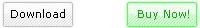Search for a Product:
Excel Math to multiple cells with formulas, adding, subtracting, multiplying, dividing and rounding functions Software! Get it now! Only \$29.95 U.S. (Regular \$49.95 US!) for a limited time!

Excel Math to multiple cells with formulas, adding, subtracting, multiplying, dividing and rounding functions --

Easily apply math functions to multiple cells with a click of a button! With this software, you can:

• Select a block of cells to apply math functions to
• Choose what value you want to multiply each cell by, divide each cell by, subtract each cell by, or add to each cell (+, -, *, /, rounding)
• Apply standard rounding functions to round up/down a block of cells at once
• Plus, the ability to specify a math formula to apply to each cell, using 'x' as a place holder for the existing cell value!

You can order by credit card, Phone/Fax, Mail, PayPal or purchase orders!

Purchase it now! Only \$29.95 U.S. for a limited time!Tutorial Video! (Click to View)Click above for a larger screenshot!